# How to Find Standard Deviation On A Calculator

If you’re taking an introductory statistics course, you’ll find out sooner than later that there’s no need for you to calculate standard deviation by hand all the time.

While some statisticians-in-training get access to computers in class and use R or SAS to calculate standard deviation, many don’t have that luxury.

But luckily, graphing calculators are a powerful alternative to computers and can help with many statistical calculations – including finding the standard deviation.

Here’s a comprehensive guide to calculating standard deviation with calculators such as the TI83 and TI84.

# How to Find Standard Deviation on Calculator

We’ve broken the process of finding standard deviation down into five easy steps.

We will explain how to find the standard deviation on a scientific calculator with an example. Consider the made-up data set: 6, 2, 7, 9, 6, 2, 3.

You don’t have to worry about calculating the population standard deviation and the sample standard deviation separately. Using this method, you will calculate both and use whatever result is relevant to you.

But before getting into how to use the deviation calculator, here’s a quick refresher of the concept so you understand precisely what you’re calculating.

## Standard Deviation: Brief Refresher

In statistics, the standard deviation measures the dispersion between the values in a data set. A lower standard deviation indicates that the data points are closer to the mean (denoted by “μ”) in the collection of data.

On the other hand, a high standard deviation means that the values in the data set are dispersed widely and aren’t very close to the mean value of the data set.

Standard deviation is denoted by the symbol “σ,” and the concept can be applied in various situations, and consequently, a variety of equations.

The formula for calculating the σ for a sample is: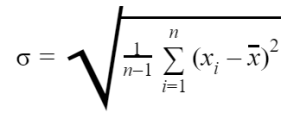Where “n” represents the sample sizes, and x represents the sample mean.

The formula for calculating the σ for a sample is: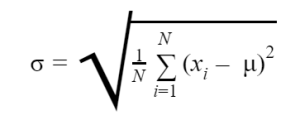Where “N” represents the population size, and represents the population mean.

Besides being used to gauge the population’s variability, standard deviation calculations are also used to measure the margin of error. When the concept is applied this way, the σ is referred to as the standard error of the mean.

## Step #1: Navigate to the Menu to Enter Data

Every time you want to find the standard deviation of a data set, you will first need to feed the data set into the calculator.

To get to the menu where you can enter the data, press the STAT button on the calculator. You will find it on the third column of keys on the TI-83 and TI-84.

You will see some options pop up. You must navigate to the “Edit” option by pressing the “1” key on the calculator.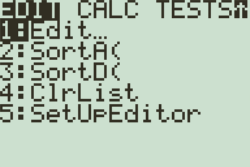Lists will appear on the screen, labeled L1 through L6.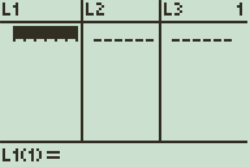### Clearing Existing Data from Lists

If there are already some data in the lists, don’t worry – it is easy to remove them. All you have to do is execute the following steps:

1. Navigate to the list you want to remove data from using the arrow keys.
2. Press the “CLEAR” button.
3. Press the “ENTER” button.
4. Repeat the steps to remove data from other lists.

## Step #2:  Enter Data into the List

Entering the data is straightforward. All you have to do is enter the number in the dataset, and hit ENTER to add it to the list.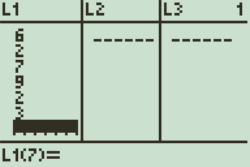Ensure you enter all of the numbers in the dataset in the same list (L1 in this example).

Note

The TI-84 and TI-83 allow you to enter data in up to six different lists. In other words, you can work with six distinct data sets with these calculators.

## Step #3: Switch to the CALC Tab

The CALC tab is the second tab under the STAT menu. Pressing the right arrow key will take you to the CALC tab.

## Step #4: Calculate 1-Variable Statistics

You will notice that the “1-Var Stats” option is the first in the list under the CALC tab. Select this option by hitting the ENTER key.

To calculate the standard deviations for the data set, you must hit the ENTER key a second time.

### If You Don’t See “L1” Next to “List”

If you have the TI-84 model, there are chances that you will not see “L1” appear next to “List.”

However, there is an easy way to fix this. All you have to do is press the “2ND” button and then press the “1” key to select “L1.”

It is also possible for you to select a different list you’ve entered data into by pressing the number key corresponding with the list.

Some non-plus models of Texas Instruments’ calculators skip this screen entirely and display the results.

## Step #5: Find Standard Deviation

After you hit the ENTER key the second time, some results will have appeared on the calculator’s screen.

As you can see, there are two deviations listed on the screen. “Sx” represents the sample standard deviation of the data, and “σx” represents the population standard deviation of the data.

These values should be the fourth and fifth entries on the results screen, respectively.

If you were to enter the example sample data, the Sx would be equal to 2.708, and the σx will be equal to 2.507.

You will also notice some other values on the result screen. The x represents the mean or average, and Σx represents the sum of the values.

# Finding Variance After Finding the Standard Deviation

You will notice that the variance doesn’t appear in the list of results. However, you can calculate it using a simple property:

Variance= (Standard Deviation)2

In our example, the standard deviation (sample) is 2.708, so the variance will be 7.333.

# Conclusion

Calculating the standard deviation of data with a calculator is relatively easy. Now that you know what menus you need to go to, you will be able to work out the deviation of your samples and populations in a matter of seconds.

We have various comprehensive calculators that you can use online for free. You can choose from t-test calculator, graphing, matrix, the standard deviation to statistics, and scientific calculators. Check it here